• +91 9971497814
• info@interviewmaterial.com

# RD Chapter 4- Inverse Trigonometric Functions Ex-4.13 Interview Questions Answers

### Related Subjects

Question 1 :

If cos-1 (x/2) + cos-1 (y/3) = α, thenprove that 9x2 – 12xy cos α + 4y2 = 36 sin2 α

Given cos-1 (x/2)+ cos-1 (y/3) = α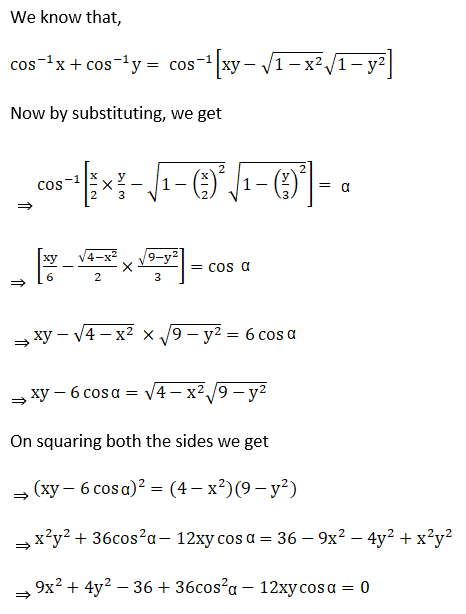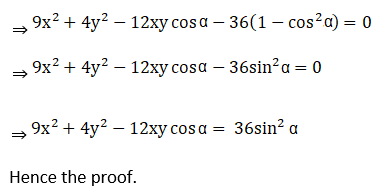Hence, proved.

Question 2 : Solve the equation: cos-1 (a/x)– cos-1 (b/x) = cos-1 (1/b) – cos-1 (1/a)

Given cos-1 (a/x)– cos-1 (b/x) = cos-1 (1/b) – cos-1 (1/a)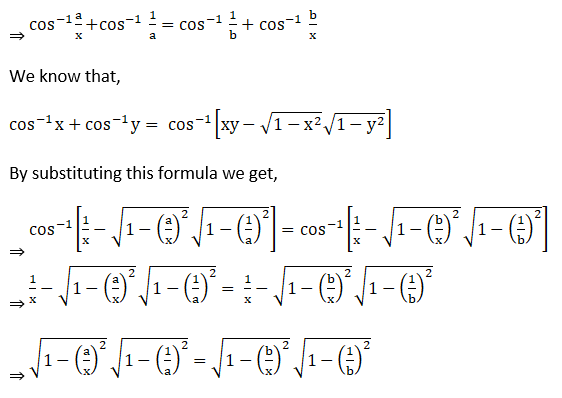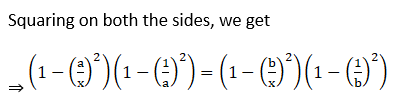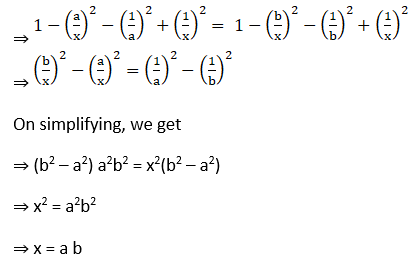Question 3 :

Solve :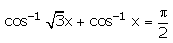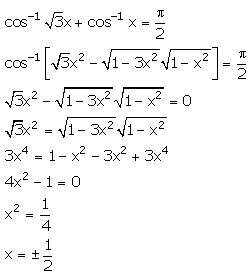Question 4 :

Prove that: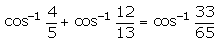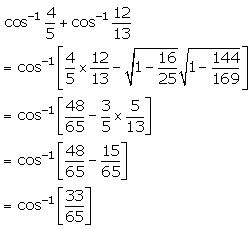Todays Deals### RD Chapter 4- Inverse Trigonometric Functions Ex-4.13 Contributorskrishan

Name:
Email:

# Latest News# 9000 interview questions in different categories